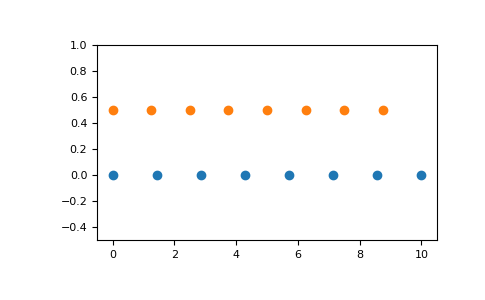# numpy.linspace¶

`numpy.``linspace`(start, stop, num=50, endpoint=True, retstep=False, dtype=None, axis=0)[source]

Return evenly spaced numbers over a specified interval.

Returns num evenly spaced samples, calculated over the interval [start, stop].

The endpoint of the interval can optionally be excluded.

Changed in version 1.16.0: Non-scalar start and stop are now supported.

Parameters: start : array_like The starting value of the sequence. stop : array_like The end value of the sequence, unless endpoint is set to False. In that case, the sequence consists of all but the last of `num + 1` evenly spaced samples, so that stop is excluded. Note that the step size changes when endpoint is False. num : int, optional Number of samples to generate. Default is 50. Must be non-negative. endpoint : bool, optional If True, stop is the last sample. Otherwise, it is not included. Default is True. retstep : bool, optional If True, return (samples, step), where step is the spacing between samples. dtype : dtype, optional The type of the output array. If `dtype` is not given, infer the data type from the other input arguments. New in version 1.9.0. axis : int, optional The axis in the result to store the samples. Relevant only if start or stop are array-like. By default (0), the samples will be along a new axis inserted at the beginning. Use -1 to get an axis at the end. New in version 1.16.0. samples : ndarray There are num equally spaced samples in the closed interval `[start, stop]` or the half-open interval `[start, stop)` (depending on whether endpoint is True or False). step : float, optional Only returned if retstep is True Size of spacing between samples.

`arange`
Similar to `linspace`, but uses a step size (instead of the number of samples).
`geomspace`
Similar to `linspace`, but with numbers spaced evenly on a log scale (a geometric progression).
`logspace`
Similar to `geomspace`, but with the end points specified as logarithms.

Examples

```>>> np.linspace(2.0, 3.0, num=5)
array([2.  , 2.25, 2.5 , 2.75, 3.  ])
>>> np.linspace(2.0, 3.0, num=5, endpoint=False)
array([2. ,  2.2,  2.4,  2.6,  2.8])
>>> np.linspace(2.0, 3.0, num=5, retstep=True)
(array([2.  ,  2.25,  2.5 ,  2.75,  3.  ]), 0.25)
```

Graphical illustration:

```>>> import matplotlib.pyplot as plt
>>> N = 8
>>> y = np.zeros(N)
>>> x1 = np.linspace(0, 10, N, endpoint=True)
>>> x2 = np.linspace(0, 10, N, endpoint=False)
>>> plt.plot(x1, y, 'o')
[<matplotlib.lines.Line2D object at 0x...>]
>>> plt.plot(x2, y + 0.5, 'o')
[<matplotlib.lines.Line2D object at 0x...>]
>>> plt.ylim([-0.5, 1])
(-0.5, 1)
>>> plt.show()
```numpy.arange

numpy.logspace